# CLASS 10 MATH NCERT SOLUTIONS FOR CHAPTER – 14 STATISTICS EX – 14.3

## Statistics

Question 1.
The following frequency distribution gives the monthly consumption of electricity of 68 consumers of a locality. Find the median, mean and mode of the data and compare them.

Solution:
Here h = 20.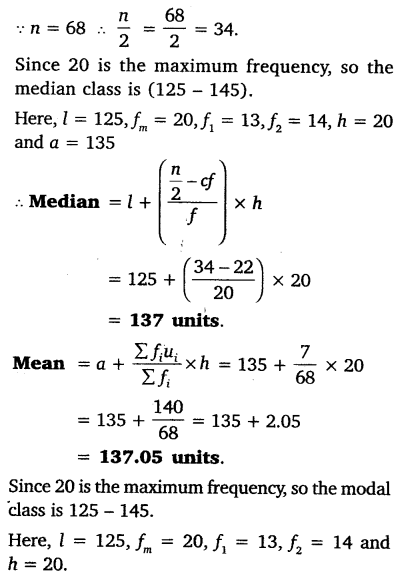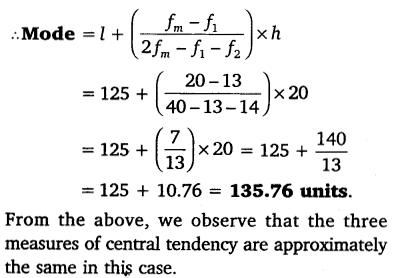Question 2.
If the median of the distribution given below is 28.5, find the values of x and y.

Solution:Question 3.
A life insurance agent found the following data for the distribution of ages of 100 policy holders. Calculate the median age, if policies are given only to persons having age 18 years onwards but less than 60 years.

Solution:
The given distribution is less than type and upper limits are 20, 25, 30, 35, 40, 45, 50, 55 and 60.
So, we can assume class intervals such as (15 – 20), (20 – 25), (25 – 30), (30 – 35), (35 – 40), (40 – 45), (45 – 50), (50 – 55) and (55 – 60). As the number of policy holder is in cumulative distribution. So have the frequency distribution as:Question 4.
The lengths of 40 leaves of a plant are measured correct to the nearest millimeter, and the data obtained is represented in the following table:

Find the median length of the leaves.

Solution: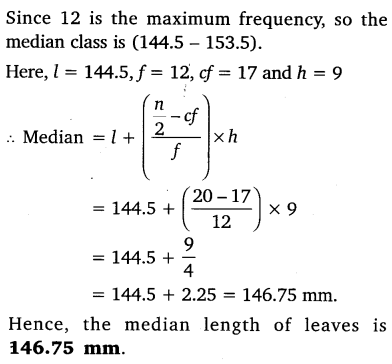Question 5.
The following table gives the distribution of the lifetime of 400 neon lamps:

Find the median lifetime of a lamp.

Solution:Question 6.
100 surnames were randomly picked up from a local telephone directory and the frequency distribution of the number of letters in the English alphabet in the surnames was obtained as follows:

Determine the median number of letters in the surnames. Find the mean number of letters in the surnames. Also, find the modal size of the surnames.

Solution: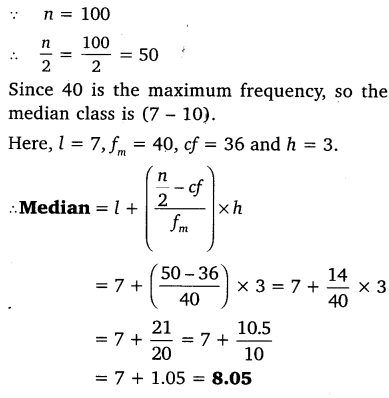Question 7.
The distribution below gives the weight of 30 students in a class. Find the median weight of the students.

Solution: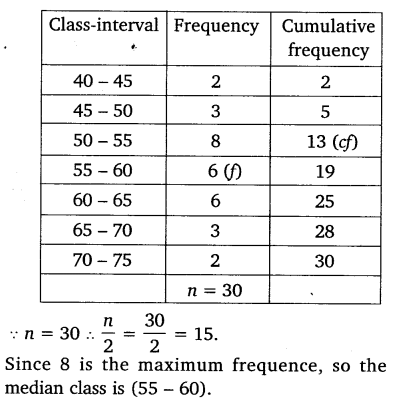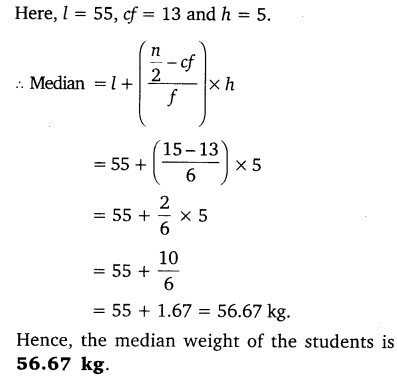#### CLASS 10 MATH NCERT SOLUTIONS FOR CHAPTER – 14 STATISTICS EX – 14.4

Lorem ipsum dolor sit amet, consectetur adipiscing elit. Phasellus cursus rutrum est nec suscipit. Ut et ultrices nisi. Vivamus id nisl ligula. Nulla sed iaculis ipsum.

Company Name